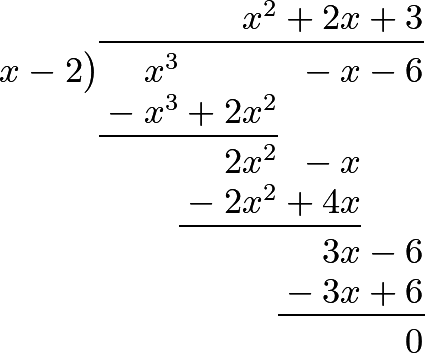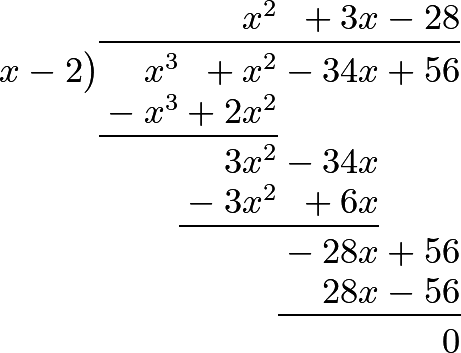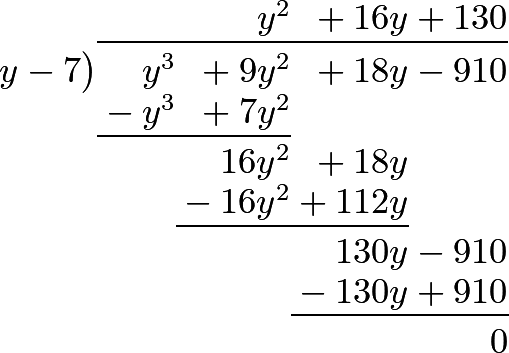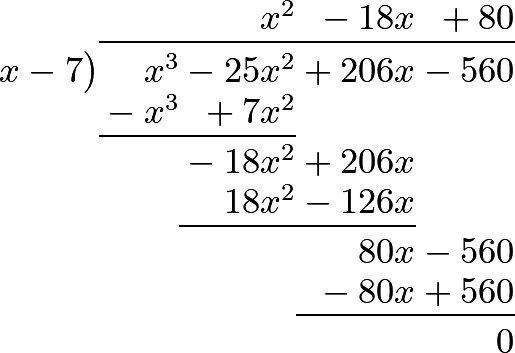### Part I. Section IV. Chapter 11. “Of the Resolution of Complete Equations of the Third Degree.”

719 An equation of the third degree is called complete, when, beside the cube of the unknown quantity, it contains that unknown quantity itself, and its square: so that the general formula for these equations, bringing all the terms to one side, is

$ax^3 \pm bx^2 \pm cx \pm d = 0.$

And the purpose of this chapter is to show how we are to derive from such equations the values of $$x$$, which are also called the roots of the equation. We suppose, in the first place, that every such an equation has three roots; since it has been seen, in the last chapter, that this is true even with regard to pure equations of the same degree.

720 We shall first consider the equation $$x^3-6x^2+11x-6=0$$; and, since an equation of the second degree may be considered as the product of two factors, we may also represent an equation of the third degree by the product of three tactors, which are in the present instance,

$(x-1)(x-2)(x-3)=0;$

since, by actually multiplying them, we obtain the given equation; for $$(x-1)(x-2)$$ gives $$x^2-3x+2$$, and multiplying this by $$x-3$$, we obtain $$x^3-6x^2+11x-6$$, which are the given quantities, and which must be =0. Now, this happens, when the product $$(x-1)(x-2)(x-3)=0$$; and, as it is sufficient for this purpose, that one of the factors become =0, three different cases may give this result, namely, when $$x-1=0$$, or $$x=1$$; secondly, when $$x-2=0$$, or $$x=2$$; and thirdly, when $$x-3=0$$, or $$x=3$$.

We see immediately also, that if we substituted for $$x$$, any number whatever beside one of the above three, none of the three factors would become equal to 0; and, consequently, the product would no longer be 0: which proves that our equation can have no other root than these three.

721 If it were possible, in every other case, to assign the three factors of such an equation in the same manner, we should immediately have its three roots. Let us, therefore, consider, in a more general manner, these three factors, $$x-p$$, $$x-q$$, $$x-r$$. Now, if we seek their product, the first, multiplied by the second, gives $$x^2-(p+q)x+pq$$, and this product, multiplied by $$x-r$$, makes

$x^3-(p+q+r)x^2+(pq+pr+qr)x-pqr.$

Hence, if this formula must become =0, it may happen in three cases: the first is that in, in which $$x-p=0$$, or $$x=p$$; and second is, when $$x-q=0$$, or $$x=q$$; the third is, when $$x-r=0$$, or $$x=r$$.

722 Let us now represent the quantity found, by the equation $$x^3-ax^2+bx-c=0$$. It is evident, in order that its three roots may be $$x=p$$, $$x=q$$, $$x=r$$, that we must have,

1. $$a=p+q+r$$,
2. $$b=pq+pr+qr$$, and
3. $$c=pqr$$.

We perceive, from this, that the second term of the equation contains the sum of the three roots; that the third term contains the sum of the products of the roots taken two by two; and lastly, that the fourth term consists of the product of all the three roots multiplied together.

From this last property we may deduce an important truth, which is, that an equation of the third degree can have no other rational roots than the divisors of the last term; for, since that term is the product of the three roots, it must be divisible by each of them: so that when we wish to find a root by trial, we immediately see what numbers we are to use.

For example, let us consider the equation, $$x^3=x+6$$, or $$x^3-x-6=0$$. Now, as this equation can have no other rational roots than numbers which are factors of the last term 6, we have only 1, 2, 3, 6, to try with, and the result of these trials will be as follows:

If $$x=1$$, we have 1 - 1 - 6 = -6.
If $$x=2$$, we have 8 - 2 -6 = 0.
If $$x=3$$, we have 26 - 3 - 6 = 18.
If $$x=6$$, we have 216 - 6 - 6 = 204.

Hence we see, that $$x=2$$ is one of the roots of the given equation; and, showing this, it is easy to find the other two; for $$x=2$$ being one of the roots $$x-2$$ is a factor of the equation, adn as we have only to see the other factor by means of division as follows:Since, therefore, the formula is represented by the product $$(x-2)(x^2+2x+3)$$, it will become =0, not only when $$x-2=0$$, or $$x=2$$, but also when $$x^2+2x+3=0$$; and, this last factor gives $$x^2+2x=-3$$; consequently, $$x=-1 \pm \surd -2$$; and these are the other two roots of our equation, which are evidently impossible, or imaginary.

723 The method which we have explained, is applicable only when the first term $$x^3$$ is multiplied by 1, and the other terms of the equation have integer coefficients; therefore, when this is not the case, we must begin by a preparation, which consists in transforming the equation into another form having the condition required; after which, we make the trial that has been already mentioned.

Let there be given, for example, the equation

$x^3-3x^2+\frac{11}{4}x-\frac{3}{4}=0;$

as it contains fourth parts, let us make $$x=\dfrac{y}{2}$$, which will give

$\dfrac{y^3}{8}-\dfrac{3y^2}{4}+\dfrac{11y}{8}-\dfrac{3}{4}=0,$

and, multiplying by 8, we shall obtain the equation

$y^3-6y^2+11y-6=0,$

the roots of which are, as we have already seen, $$y=1$$, $$y=2$$, $$y=3$$; whence it follows, that in the given equation, we have $$x=\frac{1}{2}$$, $$x=1$$, $$x=\frac{3}{2}$$.

724 Let there be an equation, where the coefficient of the first term is a whole number but not 1, and whose last term is 1; for example,

$6x^3-11x^2+6x-1=0.$

Here, if we divide by 6, we shall have $$x^3-\frac{11}{6}x^2+x-\frac{1}{6}=0$$; which equation we may clear of fractions, by the method just explained.

First, by making $$x=\dfrac{y}{6}$$, we shall have

$\dfrac{y^3}{216}-\dfrac{11y^2}{216}+\dfrac{y}{6}-\dfrac{1}{6}=0;$

and multiplying by 216, the equation will become

$y^3-11y^2+36y-36=0.$

But as it would be tedious to make trial of all the divisors of the number 36, and as the last term of the original equation is 1, it is better to suppose, in this equation, $$x=\dfrac{1}{z}$$; for we shall then have $$\dfrac{6}{z^3}-\dfrac{11}{z^2}+\dfrac{6}{z}-1=0$$, which, multiplied by $$z^2$$, gives $$6-11z+6z^2-z^3=0$$, and transposing all the terms,

$z^3-6z^2+11z-6=0;$

where the roots are $$z=1$$, $$z=2$$, $$z=3$$; whence it follows that in our equation $$x=1$$, $$x=\frac{1}{2}$$, $$x=\frac{1}{3}$$.

725 It has been observed in the preceding articles, that in order to have all the roots in positive numbers, the signs plus and minus must succeed each other alternately; by means of which the equation takes this form, $$x^3-ax^2+bx-c=0$$, the signs changing as many times as there are positive roots. If all the three roots had been negative, and we had multiplied together the three factors $$x+p$$, $$x+q$$, $$x+r$$, all the terms would have had the sign plus, and the form of the equation would have been $$x^3+ax^2+bx+c=0$$, in which the same signs follow each other three times; that is, the number of negative roots.

We may conclude, therefore, that as often as the signs change, the equation has positive roots; and that as often as the same signs follow each other, the equation has negative roots. This remark is very important, because it teaches us whether the divisors of the last term are to be taken affirmatively or negatively, when we wish to make the trial which has been mentioned.

726 In order to illustrate what has been said by an example, let us consider the equation $$x^3+x^2-34x+56=0$$, in which the signs are changed twice, and in which the same sign returns but once. Here we conclude that the equation has two positive roots, and one negative root; and as these roots must be divisors of the last terra 56, they must be included in the numbers ±1, 2, 4, 7, 8, 14, 28, 56.

Let us, therefore, make $$x=2$$, and we shall have $$8+4-68+56=0$$; whence we conclude that $$x=2$$ is a positive root, and that therefore $$x-2$$ is a divisor of the equation, by means of which we easily find the two other roots; for, actually dividing by $$x-2$$, we haveAnd making the quotient $$x^2-3x-28=0$$, we find the two other roots; which will be

$x=-\frac{3}{2} \pm \surd\left(\frac{9}{4}+24\right)=-\frac{3}{2} \pm \frac{11}{2};$

that is, $$x=4$$; or $$x=-7$$; and taking into account the root found before, namely, $$x=2$$, we clearly perceive that the equation has two positive, and one negative root. We shall give some examples to render this still more evident.

727 Question 1. There are two numbers, whose difference is 12, and whose product multiplied by their sum makes 14560. What are those numbers?

Let $$x$$ be the less of the two numbers, then the greater will be $$x+12$$, and their product will be $$x^2+12x$$, which multiplied by the sum $$2x+12$$, gives

$2x^3+36x^2+144x=14560;$

and dividing by 2, we have

$x^3+18x^2+72x=7280.$

Now, the last term 7280 is too great for us to make trial of all its divisors; but as it is divisible by 8, we shall make $$x=2y$$, because the new equation, $$8y^3+72y^2+144y=7280$$, after the substitution, being divided by 8, will become

$y^3+9y^2+18y=910;$

to solve which, we need only try the divisors 1, 2, 5, 7, 10, 13, etc. of the number 910: where it is evident, that the three first, 1, 2, 5, are too small; beginning therefore with supposing $$y=7$$, we immediately find that number to be one of the roots; for the substitution gives

343 + 144 + 126 = 910.

It follows, therefore, that $$x=14$$; and the two other roots will be found by dividing $$y^3+9y^2+18y-910$$ by $$y-7$$, thus:Supposing now this quotient $$y^2+16y+130=0$$, we shall have $$y^2 + 16y = -130$$, and thence $$y=-8 \pm \surd -66$$; a proof that the other two roots are impossible.

The two numbers sought are therefore 14, and (14 + 12) = 26; the product of which, 364, multiplied by their sum, 40, gives 14560.

728 Questlon 2. To find two numbers whose difference is 18, and such, that their sum multiplied by the difference of their cubes, may produce 275184.

Let $$x$$ be the less of the two numbers, then $$x+18$$ will be the greater; the cube of the first will be $$x^3$$, and the cube of the second $$x^3+5x^2+972x+5832$$; the difference of the cubes

$54x^2+972x+5832=54(x^2+18x+108),$

which multiplied by the sum $$2x+18$$, or $$2(x+9)$$, gives the product

$108(x^3+27x^2+270x+972)=275184.$

And, dividing by 108, we have

$x^3+27x^2+270x+972=2548,$

or

$x^3+27x^2+270x=1576.$

Now, the divisors of 1576 are 1, 2, 4, 8, etc. the two first of which are too small; but if we try $$x = 4$$, that number is found to satisfy the terms of the equation.

It remains, therefore, to divide by $$x-4$$, in order to find the two other roots; which division gives the quotient $$x^2+31x+394$$; making therefore

$x^2+31x=-394,$

we shall find

$x=-\frac{31}{2} \pm \surd\left(\frac{961}{4}-\frac{1376}{4} \right);$

that is, two imaginary roots.

Hence the numbers sought are 4, and (4 + 18) = 22.

729 Question 3. Required two numbers whose difference is 720, and such, that if the less be multiplied by the square root of the greater, the product may be 20736.

If the less be represented by $$x$$, the greater will evidently be $$x+720$$; and, by the question,

$x \surd(x+720) = 20736 = 8\cdot 8\cdot 4\cdot 81.$

Squaring both sides, we have

$x^2(x+720) = x^3+720x^2 = 8^2 \cdot 8^2\cdot 4^2\cdot 81^2.$

Let us now make $$x=8y$$; this supposition gives

$8^3 y^3 + 720 \cdot 8^2 y^2 = 8^2 \cdot 8^2\cdot 4^2\cdot 81^2;$

and dividing by $$8^3$$, we have $$y^3+90y^2=8\cdot 4^2\cdot 81^2$$. Farther, let us suppose $$y=2z$$, and we shall have $$8z^3+4\cdot 90z^2 = 8\cdot 4^2\cdot 81^2$$; or, dividing by 8,

$z^3+45z^2=4^2\cdot 81^2.$

Again, make $$z=9u$$, in order to have, in this last equation,

$9^3u^3+45\cdot 9^2u^2=4^2\cdot 9^4,$

because dividing now by $$9^3$$, the equation becomes

$u^3+5u^2=4^2\cdot 9,$

or

$u^2(u+5)=16\cdot 9 = 144;$

where it is obvious, that $$u=4$$; for in this case $$u^2=16$$, and $$u+5=9$$: since, therefore, $$u=4$$, we have

$z=36, \qquad y=72, \quad \textrm{and} \quad x=576,$

which is the less of the two numbers sought; so that the greater is 1296, and the square root of this last, or 36, multiplied by the other number 576, gives 20736.

730 Remark. This question admits of a simpler solution; for since the square root of the greater number, multiplied by the less, must give a product equal to a given number, the greater of the two numbers must be a square. If, therefore, from this consideration, we suppose it to be $$x^2$$, the other number will be $$x^2-720$$, which being multiplied by the square root of the greater, or by $$x$$, we have

$x^3-720x=20736=64\cdot 27\cdot 12$

If we make $$x=4y$$, we shall have

$64y^3-720\cdot 4y=64\cdot 27\cdot 12,$

or

$y^3-45y^2=27\cdot 12$

Supposing, farther, $$y=3z$$, we find $$27z^3-135z=27\cdot 12$$; or, dividing by 27, $$z^3-5z=12$$, or

$z^3-5z-12=0.$

The divisors of 12 are 1, 2, 3, 4, 6, 12: the first two are too small; but the supposition of $$z=3$$ gives exactly 27 - 15 - 12 = 0. Consequently,

$z=3, \qquad y=9, \quad \textrm{and} \quad x=36;$

whence we conclude, that the greater of the two numbers sought, or $$x^2$$, =1296, and that the less, or $$x^2-720$$, =576, as before.

731 Question 4. There are two numbers, whose difference is 12; the product of this difference by the sum of their cubes is 102144; what are the numbers?

Calling the less of the two numbers $$x$$, the greater will be $$x+12$$; also the cube of the first is $$x^3$$, and of the second $$x^3+36x^2+432x+1728$$; the product also of the sum of these cubes by the difference 12, is

$12(2x^3+36x^2+423x+1728)=102144;$

and, dividing successively by 12 and by 2, we have

$x^3+18x^2+216x+864=4256,$

or

$x^3+18x^2+216x=3392=8\cdot 8\cdot 53.$

If now we substitute $$x=2y$$, and divide by 8, we shall have

$y^3+9y^2+54y=8\cdot 53=424.$

Now, the divisors of 424 are 1, 2, 4, 8, 53, etc. 1 and 2 are evidently too small; but if we make $$y=4$$, we find 64+144+216=424. So that $$y=4$$, and $$x=8$$; whence we conclude that the two numbers sought are 8 and (8+12)=20.

732 Question 5. Several persons form a partnership, and establish a certain capital, to which each contributes ten times as many pounds as there are persons in company: they gain 6 plus the number of partners per cent; and the whole profit is 392 pounds: required how many partners there are?

Let $$x$$ be the number required ; then each partner will have furnished $$10x$$ pounds, and conjointly $$10x^2$$ pounds; and since they gain $$x+6$$ per cent, they will have gained with the whole capital, $$\dfrac{x^3+6x^2}{10}$$, which is to be made equal to 392.

We have, therefore, $$x^3+6x^2=3920$$, consequently, making $$x=2y$$, and dividing by 8, we have

$y^3+3y^2=490.$

Now, the divisors of 490 are 1, 2, 5, 7, 10, etc. the first three of which are too small; but if we suppose $$y=7$$, we have 343 + 147 = 490; so that $$y=7$$, and $$x=14$$.

There are therefore fourteen partners, and each of them put 140 pounds into the common stock.

733 Question 6. A company of merchants have a common stock of 8240 pounds; and each contributes to it forty times as many pounds as there are partners; with which they gain as much per cent as there are partners: now, on dividing the profit, it is found, after each has received ten times as many pounds as there are persons in the company, that there still remains 224l.. Required the number of merchants?

If $$x$$ be made to represent the number, each will have contributed $$40x$$ to the stock; consequently, all together will have contributed $$40x^2$$, which makes the stock $$=40x^2+8240$$. Now, with this sum they gain $$x$$ per cent; so that the whole gain is

$\dfrac{40x^3}{100}+\dfrac{8240x^2}{100} = \frac{4}{10}x^3+\frac{824}{10}x^2 = \frac{2}{5}x^3+\frac{412}{5}x^2.$

From which sum each receives $$10x$$, and consequently they all together receive $$10x^2$$, leaving a remainder of 224; the profit must therefore have been $$10x^2+224$$, and we have the equation

$\frac{2}{5}x^3+\frac{412}{5}x^2=10x^2+224.$

Multiplying by 5 and dividing by 2, we have

$x^3+206x=25x^2+560,$

or

$x^3-25x^2+206x-560=0:$

the first, however, will be more convenient for trial. Here the divisors of the last term are 1, 2, 4, 5, 7, 8, 10, 14, 16, etc. and they must be taken positively; because in the second form of the equation the signs vary three times, which shows that all the three roots are positive.

Here, if we first try $$x=1$$, and $$x=2$$, it is evident that the first side will become less than the second. We shall therefore make trial of other divisors.

When $$x=4$$, we have 64 + 824 = 400 + 560, which does not satisfy the terms of the equation.

If $$x=5$$, we have 125 + 1030 = 625 + 560, which likewise does not succeed.

But if $$x = 7$$, we we have 343 + 1442 = 1225 + 560, which answers to the equation; so that $$x=7$$ is a root of it. Let us now seek for the other two, by dividing the second form of our equation by $$x-7$$.Now, making this quotient equal to nothing, we have $$x^2-18x+80=0$$, or $$x^2-18x=-80$$; which gives $$x=9 \pm 1$$, so that the two other roots are $$x=8$$; or $$x=10$$.

This question therefore admits of three answers. According to the first, the number of merchants is 7; according to the second, it is 8; and, according to following statement shows, that all these will answer the conditions of the question:

 Number of merchants 7 8 10 Each contributes $$40x$$ 280 320 400 In all they contribute $$40x^2$$ 1960 2560 4000 The original stock was 8240 8240 8240 The whole stock is $$40x^2+8240$$ 10200 10800 12240 With this capital they gain as much per cent as there are partners 714 864 1224 Each takes from it 70 80 100 So that they all together take $$10x^2$$ 490 640 1000 Therefore there remains 224 224 224

#### Editions

1. Leonhard Euler. Elements of Algebra. Translated by Rev. John Hewlett. Third Edition. Longmans, Hurst, Rees, Orme, and Co. London. 1822.
2. Leonhard Euler. Vollständige Anleitung zur Algebra. Mit den Zusätzen von Joseph Louis Lagrange. Herausgegeben von Heinrich Weber. B. G. Teubner. Leipzig and Berlin. 1911. Leonhardi Euleri Opera omnia. Series prima. Opera mathematica. Volumen primum.